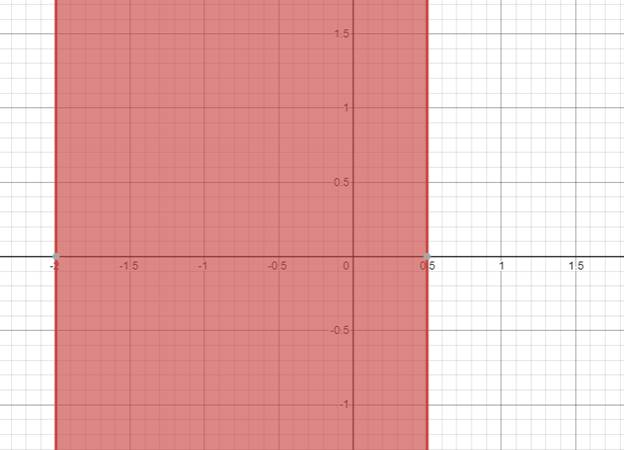# The solution of given nonlinear inequality equation and plot a graph for it.### Precalculus: Mathematics for Calcu...

6th Edition
Stewart + 5 others
Publisher: Cengage Learning
ISBN: 9780840068071### Precalculus: Mathematics for Calcu...

6th Edition
Stewart + 5 others
Publisher: Cengage Learning
ISBN: 9780840068071

#### Solutions

Chapter 1.7, Problem 44E
To determine

## The solution of given nonlinear inequality equation and plot a graph for it.

Expert Solution

2x0.5

### Explanation of Solution

Given:

Non-linear inequality equation

5x2+3x3x2+2

Calculation,

Non-linear inequality equation,

5x2+3x3x2+25x23x2+3x202x2+3x202x2+(41)x202x2+4xx202x(x+2)1(x+2)0(2x1)(x+2)0Now,2x102x1x12x0.5and,x+20x2so,2x0.5

Now, graph of 5x2+3x3x2+2Conclusion:

Hence, the values of x lies between the -2 and 0.5.

### Have a homework question?

Subscribe to bartleby learn! Ask subject matter experts 30 homework questions each month. Plus, you’ll have access to millions of step-by-step textbook answers!